Schedule View>  Feature/Function: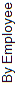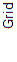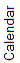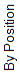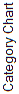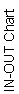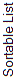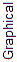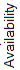Daily View x x x x x x x x Weekly View x x x x 1-4 wks 1-4 wks x x x x Multiple Weekly View x x Monthly View x x x x Add Shifts x x x x x x x x x x Edit (& Assign) Shifts x x x x x x x x x x DRAG & DROP Shifts (& UNDO) x QUICK EDIT & Add Shifts x x View Time Off on Schedule x Edit Multiple Shifts x x Bulk Edit x Put Multiple Shifts Into Categories x x Prevent page refreshing x x x x x x x x x x Delete Shifts by Clicking Shift x x x x x x x x x Delete Multiple Shifts x x Unassign Shifts by Clicking Shift x x x x x x x x x Unassign Multiple Shifts x x Shifts display by Category Name x x x Sort Shifts by Column x x x Sort/ Group View by Category x Weekly-Monthly x Search for Shifts x x x x x x x x x Filter View by Category x x x x x x x x x x x Filter View by Position x x x x x x x x x x x Filter to show Autofilled, Unassigned, & Preplaced Shifts x x x Save Template x x x x x x x x x Import (Unpublished views) x x Daily-Weekly x x x x x x Export x x Daily-Weekly x x x x x x Export To Clipboard x Unpublish / Publish x x Daily-Weekly x x x x x x Print x x x x x x x x x x x Add Weekly Notes x x Daily-Weekly x x x x x Employee Phone # on Schedule x x Daily Unassigned shifts on Schedule x x x x x x x x x See which employees have viewed/confirmed schedule x x Print Individual Employee’s Weekly Schedules (Individual Monthly use Employee Details Schedule tab) x x See employee preferences superimposed on shifts x Controls How Employees’ Everyone’s Schedule displays x x x Analyze Shifts – show conflicts x x x Show Hours Over Monthly Target Monthly Show Overtime (hours over Maximum) x x